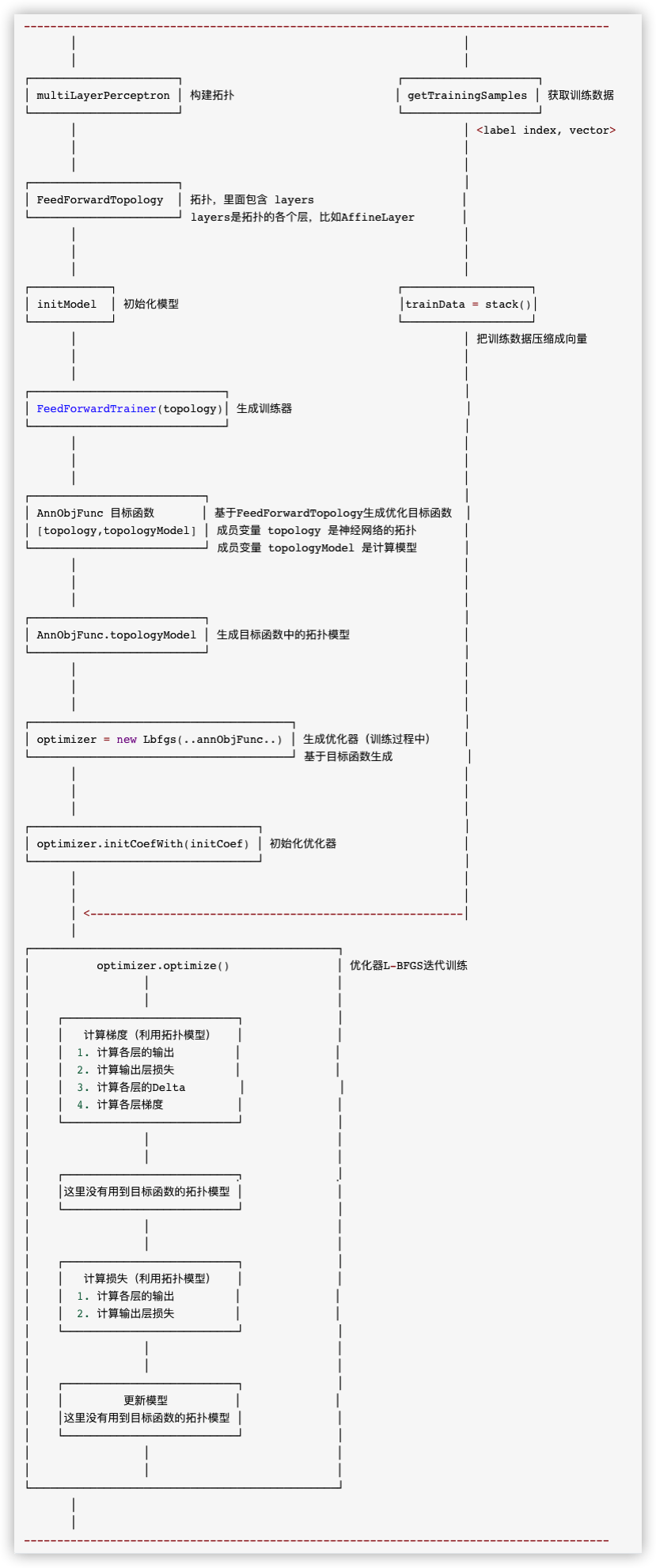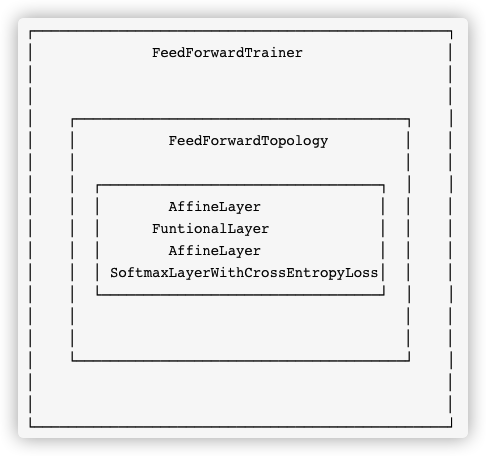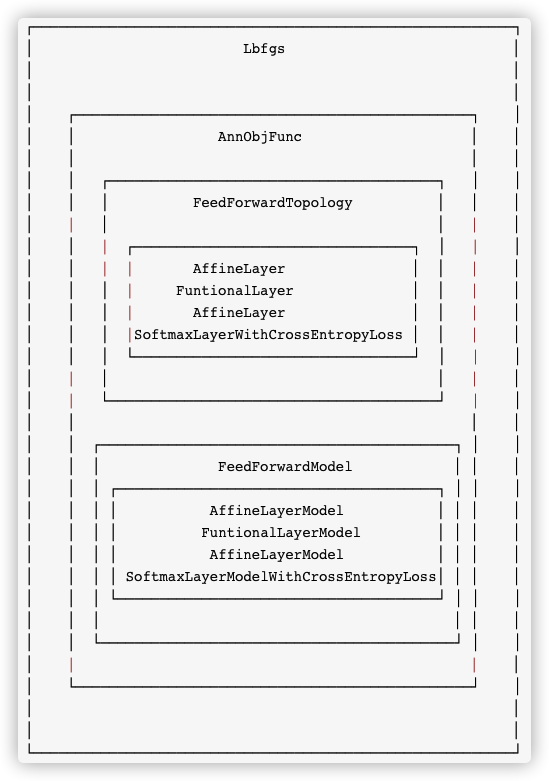• 0x00 摘要
• 0x01 背景概念
• 1.1 前馈神经网络
• 1.2 反向传播
• 1.3 代价函数
• 1.4 优化过程
• 1.4.1 迭代法
• 1.4.2 梯度下降
• 1.5 相关公式
• 1.5.1 加权求和 h
• 1.5.2 神经元输出值 a
• 1.5.3 输出层的输出值 y
• 1.5.4 激活函数g(h)
• 1.5.5 损失函数E
• 1.5.6 误差反向传播——更新权重
• 1.5.7 输出层增量项 δo
• 1.5.8 更新输出层权重wjk
• 0x02 示例代码
• 0x03 训练总体逻辑
• 3.1 总体逻辑示例图
• 3.2 L-BFGS训练调用逻辑概述
• 3.3 获取训练数据
• 3.4 构建拓扑
• 3.4.1 AffineLayer
• 3.4.2 FuntionalLayer
• 3.4.3 SoftmaxLayerWithCrossEntropyLoss
• 3.4.3.1 Softmax
• 3.4.3.2 softmax loss
• 3.4.3.3 cross entropy
• 3.4.3.4 SoftmaxLayerWithCrossEntropyLoss
• 3.5 构建训练器
• 0xFF 参考

## 0x01 背景概念

### 1.2 反向传播

• 代价信息如何传递到深度模型的每一层？
• 基于传递到本层的信息，本层的参数应该如何更新？

### 1.3 代价函数

• 代价函数必须能够表达为平均值。

• 代价函数不能依赖于输出层旁边网络的任何激活值。

### 1.4 优化过程

#### 1.4.2 梯度下降

[x_{n+1} = x_n – ηfrac{df(x)}{dx} ]

### 1.5 相关公式

#### 1.5.1 加权求和 h

[h_j = sum_{i=0}^M w_{ij}x_j ]

hj 表示当前节点的所有输入加权之和。

#### 1.5.2 神经元输出值 a

[a_j = g(h_j) = g(sum_{i=0}^M w_{ij}x_j) ]

• a_j 表示隐藏层神经元的输出值。
• g()代表激活函数，w是权重，x是输入。
• a_j=x_jk 即当前层神经元的输出值，等于下一层神经元的输入值。

#### 1.5.3 输出层的输出值 y

[y = a_k = g(h_k) = g(sum_{i=0}^M w_{jk}x_{jk}) ]

• y 表示输出层的值，也就是最终结果。
• h_k 表示输出层神经元k的输入加权之和。

#### 1.5.4 激活函数g(h)

[g(h)=σ(h)= frac{1}{1+e^{-h}} ]

sigmoid函数的导数：

[σ ′(x)=σ(x)[1−σ(x)] ]

[g ′ (h)=a_j (1−a_j ) ]

#### 1.5.5 损失函数E

[E = frac{1}{2}sum _{k=1}^N(y-t)^2 ]

• 平方是为了避免超平面两端的误差点相互抵消（y−t 存在正负）。
• 前面系数取1/2 是为了之后采用梯度下降时，求梯度（偏导数）时能抵消平方求导后的2。

#### 1.5.6 误差反向传播——更新权重

[frac{∂E}{∂w_{ik}} = frac{∂E}{∂h_k} frac{∂h_k}{∂w_{ik}} ]

h_k表示输出层神经元k的所有输入加权之和，也就是激活函数g(h)的输入值。

#### 1.5.7 输出层增量项 δo

[δ_o(k) = frac{∂E}{∂h_{k}} = frac{∂E}{∂y} frac{∂y}{∂h_k} = (y−t)g ′(h_ k ) ]

#### 1.5.8 更新输出层权重wjk

[w_{jk} ←w _{jk} −η frac{∂E}{∂w_{jk}} ]

[w_{jk} = w_{jk} – ηδ_o(k)a_i ]

ai是上一层的输出值，也即是输出层的输入值xi。

## 0x02 示例代码

``public class MultilayerPerceptronClassifierExample {     public static void main(String[] args) throws Exception {         BatchOperator data = Iris.getBatchData();          MultilayerPerceptronClassifier classifier = new MultilayerPerceptronClassifier()                 .setFeatureCols(Iris.getFeatureColNames())                 .setLabelCol(Iris.getLabelColName())                 .setLayers(new int[]{4, 5, 3})                 .setMaxIter(100)                 .setPredictionCol("pred_label")                 .setPredictionDetailCol("pred_detail");          BatchOperator res = classifier.fit(data).transform(data);          res.print();     } } ``

Iris定义如下

``public class Iris {     final static String URL = "https://alink-release.oss-cn-beijing.aliyuncs.com/data-files/iris.csv";     final static String SCHEMA_STR             = "sepal_length double, sepal_width double, petal_length double, petal_width double, category string";      public static BatchOperator getBatchData() {         return new CsvSourceBatchOp(URL, SCHEMA_STR);     }      public static StreamOperator getStreamData() {         return new CsvSourceStreamOp(URL, SCHEMA_STR);     }      public static String getLabelColName() {         return "category";     }      public static String[] getFeatureColNames() {         return new String[] {"sepal_length", "sepal_width", "petal_length", "petal_width"};     } } ``

## 0x03 训练总体逻辑

MultilayerPerceptronTrainBatchOp 类是批处理训练的实现。

``protected BatchOperator train(BatchOperator in) { 	return new MultilayerPerceptronTrainBatchOp(this.getParams()).linkFrom(in); } ``

• 1）获取一些元信息，比如label名称，特征列名，特征类型等；
• 2）获取测试数据 `trainData = getTrainingSamples`
• 3）训练
• 3.1）获取初始权重 `initialWeights = getInitialWeights();`
• 3.2）构建拓扑 `topology = FeedForwardTopology.multiLayerPerceptron`
• 3.3）构建训练器 `FeedForwardTrainer`
• 3.3.1）初始化模型
• 3.3.2）构建目标函数
• 3.3.3）训练器会基于目标函数构建优化器，这里的优化器是`L-BFGS`
• 3.4）训练获取最终权重 `weights = trainer.train`
• 4）输出模型 `DataSet<Row>；`
• 5）把`DataSet<Row>`转成Table；
``@Override public MultilayerPerceptronTrainBatchOp linkFrom(BatchOperator<?>... inputs) {         BatchOperator<?> in = checkAndGetFirst(inputs);          // 1）获取一些元信息，比如label名称，特征列名，特征类型等。         final String labelColName = getLabelCol();         final String vectorColName = getVectorCol();         final boolean isVectorInput = !StringUtils.isNullOrWhitespaceOnly(vectorColName);         final String[] featureColNames = isVectorInput ? null :             (getParams().contains(FEATURE_COLS) ? getFeatureCols() :                 TableUtil.getNumericCols(in.getSchema(), new String[]{labelColName}));          final TypeInformation<?> labelType = in.getColTypes()[TableUtil.findColIndex(in.getColNames(),             labelColName)];         DataSet<Tuple2<Long, Object>> labels = getDistinctLabels(in, labelColName);  // 此处程序变量如下： labelColName = "category" vectorColName = null isVectorInput = false featureColNames = {String@6412}   0 = "sepal_length"  1 = "sepal_width"  2 = "petal_length"  3 = "petal_width" labelType = {BasicTypeInfo@6414} "String" labels = {MapOperator@6415}               // 2）获取测试数据         // get train data         DataSet<Tuple2<Double, DenseVector>> trainData =             getTrainingSamples(in, labels, featureColNames, vectorColName, labelColName);          // train 3）训练         final int[] layerSize = getLayers();         final int blockSize = getBlockSize();         // 3.1）获取初始权重         final DenseVector initialWeights = getInitialWeights();         // 3.2）获取拓扑         Topology topology = FeedForwardTopology.multiLayerPerceptron(layerSize, true);         // 3.3）构建训练器          FeedForwardTrainer trainer = new FeedForwardTrainer(topology,             layerSize, layerSize[layerSize.length - 1], true, blockSize, initialWeights);         // 3.4）训练获取最终权重          DataSet<DenseVector> weights = trainer.train(trainData, getParams());          // output model 4）输出模型         DataSet<Row> modelRows = weights             .flatMap(new RichFlatMapFunction<DenseVector, Row>() {                 @Override                 public void flatMap(DenseVector value, Collector<Row> out) throws Exception {                     List<Tuple2<Long, Object>> bcLabels = getRuntimeContext().getBroadcastVariable("labels");                     Object[] labels = new Object[bcLabels.size()];                     bcLabels.forEach(t2 -> {                         labels[t2.f0.intValue()] = t2.f1;                     });                      MlpcModelData model = new MlpcModelData(labelType);                     model.labels = Arrays.asList(labels);                     model.meta.set(ModelParamName.IS_VECTOR_INPUT, isVectorInput);                     model.meta.set(MultilayerPerceptronTrainParams.LAYERS, layerSize);                     model.meta.set(MultilayerPerceptronTrainParams.VECTOR_COL, vectorColName);                     model.meta.set(MultilayerPerceptronTrainParams.FEATURE_COLS, featureColNames);                     model.weights = value;                     new MlpcModelDataConverter(labelType).save(model, out);                 }             })             .withBroadcastSet(labels, "labels");          // 5）把DataSet<Row>转成Table         setOutput(modelRows, new MlpcModelDataConverter(labelType).getModelSchema()); } ``

### 3.1 总体逻辑示例图

``----------------------------------------------------------------------------------------        │                                                          │          │                                                          │   ┌──────────────────────┐                    		┌────────────────────┐ │ multiLayerPerceptron │ 构建拓扑                        │ getTrainingSamples │ 获取训练数据 └──────────────────────┘                                └────────────────────┘              │                                                          │ <label index, vector>         │                                                          │                  │                                                          │   ┌──────────────────────┐                                          │ │ FeedForwardTopology  │ 拓扑，里面包含 layers                      │ └──────────────────────┘ layers是拓扑的各个层，比如AffineLayer       │        │                                                          │               │                                                          │          │                                                          │       ┌────────────┐                    		        ┌────────────────────┐ │ initModel  │ 初始化模型                                │trainData = stack() │ └────────────┘                                          └────────────────────┘            │                                                          │ 把训练数据压缩成向量             │                                                          │          │                                                          │    ┌─────────────────────────────┐                                   │ │ FeedForwardTrainer(topology)│ 生成训练器                          │ └─────────────────────────────┘                                   │         │                                                          │            │                                                          │             │                                                          │        ┌──────────────────────────┐                                      │ │ AnnObjFunc 目标函数       │ 基于FeedForwardTopology生成优化目标函数  │ │ [topology,topologyModel] │ 成员变量 topology 是神经网络的拓扑       │ └──────────────────────────┘ 成员变量 topologyModel 是计算模型       │         │                                                          │         │                                                          │         │                                                          │     ┌──────────────────────────┐                                      │ │ AnnObjFunc.topologyModel │ 生成目标函数中的拓扑模型                 │ └──────────────────────────┘                                      │         │                                                          │           │                                                          │                 │                                                          │    ┌───────────────────────────────────────┐                         │ │ optimizer = new Lbfgs(..annObjFunc..) │ 生成优化器（训练过程中）    │ └───────────────────────────────────────┘ 基于目标函数生成           │         │                                                          │             │                                                          │           │                                                          │     ┌──────────────────────────────────┐                              │ │ optimizer.initCoefWith(initCoef) │ 初始化优化器                   │ └──────────────────────────────────┘                              │          │                                                          │             │                                                          │                 │ <--------------------------------------------------------│           │                                                            ┌──────────────────────────────────────────────┐ │          optimizer.optimize()                │ 优化器L-BFGS迭代训练 │                 │                            │  │                 │                            │    │    ┌──────────────────────────┐              │    │    │   计算梯度（利用拓扑模型）   │              │ │    │  1. 计算各层的输出         │              │ │    │  2. 计算输出层损失         │              │ │    │  3. 计算各层的Delta        │              │ │    │  4. 计算各层梯度           │              │   │    └──────────────────────────┘              │  │                 │                            │  │                 │                            │    │    ┌──────────────────────────┐              │    │    │         计算方向          │              │ │    │这里没有用到目标函数的拓扑模型 │              │ │    └──────────────────────────┘              │    │                 │                            │  │                 │                            │    │    ┌──────────────────────────┐              │    │    │   计算损失（利用拓扑模型）   │              │ │    │  1. 计算各层的输出         │              │ │    │  2. 计算输出层损失         │              │ │    └──────────────────────────┘              │  │                 │                            │  │                 │                            │    │    ┌──────────────────────────┐              │    │    │         更新模型          │              │ │    │这里没有用到目标函数的拓扑模型 │              │ │    └──────────────────────────┘              │    │                 │                            │  │                 │                            │       └──────────────────────────────────────────────┘        │                                                                       │                       ---------------------------------------------------------------------------------------- ``### 3.2 L-BFGS训练调用逻辑概述

• `CalcGradient()` 计算梯度
• `CalDirection(...)` 计算方向
• `CalcLosses(...)` 计算损失
• `UpdateModel(...)` 更新模型

• 1）调用 `AnnObjFunc.updateGradient；`
• 1.1）调用 目标函数中拓扑模型 `topologyModel.computeGradient` 来计算
• 1.1.1）计算各层的输出；`forward(data, true)`
• 1.1.2）计算输出层损失；`labelWithError.loss`
• 1.1.3）计算各层的Delta；`layerModels.get(i).computePrevDelta`
• 1.1.4）计算各层梯度；`layerModels.get(i).grad`

CalDirection 计算方向

• 这里没有用到目标函数的拓扑模型。

CalcLosses 计算损失

• 1）调用 `AnnObjFunc.calcSearchValues;` 其内部会调用 `calcLoss` 计算损失；
• 1.1）调用 `topologyModel.computeGradient` 来计算损失
• 1.1.1）计算各层的输出；`forward(data, true)`
• 1.1.2）计算输出层损失；`labelWithError.loss`

UpdateModel 更新模型

• 这里没有用到目标函数的拓扑模型。

### 3.3 获取训练数据

getTrainingSamples函数将从原始输入获取训练数据。

``5.1	3.5	1.4	0.2	Iris-setosa 5	2	3.5	1	Iris-versicolor 5.1	3.7	1.5	0.4	Iris-setosa 6.4	2.8	5.6	2.2	Iris-virginica 6	2.9	4.5	1.5	Iris-versicolor ``

• 1）获取元数据，比如特征列的index，label列的index；
• 2）把labels广播，后续会在open函数中使用；
• 3）open函数中得倒一个 `label : index` 的映射
• 4）map 函数中有两种执行序列，都会转换为 `<label index, vector>` 这样的二元组
• 4.1）原始输入中有vector，比如类似 5.1 3.5 1.4 0.2 Iris-setosa 5.1 3.5 1.4 0.2，这些加粗的就是vector。
• 4.2）原始输入中没有vector，比如类似 5.1 3.5 1.4 0.2 Iris-setosa ；

``private static DataSet<Tuple2<Double, DenseVector>> getTrainingSamples(         BatchOperator data, DataSet<Tuple2<Long, Object>> labels,         final String[] featureColNames, final String vectorColName, final String labelColName) {                  // 1）获取元数据，比如特征列的index，label列的index；         final boolean isVectorInput = !StringUtils.isNullOrWhitespaceOnly(vectorColName);         final int vectorColIdx = isVectorInput ? TableUtil.findColIndex(data.getColNames(), vectorColName) : -1;         final int[] featureColIdx = isVectorInput ? null : TableUtil.findColIndices(data.getSchema(),             featureColNames);         final int labelColIdx = TableUtil.findColIndex(data.getColNames(), labelColName);  // 程序变量如下 isVectorInput = false vectorColIdx = -1 featureColIdx = {int@6443}   0 = 0  1 = 1  2 = 2  3 = 3 labelColIdx = 4              DataSet<Row> dataRows = data.getDataSet();         return dataRows             .map(new RichMapFunction<Row, Tuple2<Double, DenseVector>>() {                 transient Map<Comparable, Long> label2index;                  @Override                 public void open(Configuration parameters) throws Exception {                     List<Tuple2<Long, Object>> bcLabels = getRuntimeContext().getBroadcastVariable("labels");                     this.label2index = new HashMap<>();                     // 得倒一个label : index 的映射                     bcLabels.forEach(t2 -> {                         Long index = t2.f0;                         Comparable label = (Comparable) t2.f1;                         this.label2index.put(label, index);                     }); // 变量是 this = {MultilayerPerceptronTrainBatchOp\$2@11578}   label2index = {HashMap@11580}  size = 3   "Iris-versicolor" -> {Long@11590} 2   "Iris-virginica" -> {Long@11592} 1   "Iris-setosa" -> {Long@11594} 0                                                          }                  @Override                 public Tuple2<Double, DenseVector> map(Row value) throws Exception {                     Comparable label = (Comparable) value.getField(labelColIdx);                     Long labelIdx = this.label2index.get(label);                      if (isVectorInput) { // 4.1）如果原始输入中有vector                         Vector vec = VectorUtil.getVector(value.getField(vectorColIdx));                         // 转换为 <label index,  vector> 这样的二元组                         if (null == vec) {                             return new Tuple2<>(labelIdx.doubleValue(), null);                         } else {                             return new Tuple2<>(labelIdx.doubleValue(),                                 (vec instanceof DenseVector) ? (DenseVector) vec                                     : ((SparseVector) vec).toDenseVector());                         }                     } else { // 4.2）如果原始输入中没有vector                         int n = featureColIdx.length;                         DenseVector features = new DenseVector(n);                         for (int i = 0; i < n; i++) {                             double v = ((Number) value.getField(featureColIdx[i])).doubleValue();                             features.set(i, v);                         }                          // 转换为 <label index,  vector> 这样的二元组                         return Tuple2.of(labelIdx.doubleValue(), features);                     }                 }             })             .withBroadcastSet(labels, "labels"); // 2）把labels广播，在open函数中使用； } ``

### 3.4 构建拓扑

`FeedForwardTopology.multiLayerPerceptron` 完成了构建前馈神经网络拓扑的工作。

``public static FeedForwardTopology multiLayerPerceptron(int[] layerSize, boolean softmaxOnTop) {         List<Layer> layers = new ArrayList<>((layerSize.length - 1) * 2);         for (int i = 0; i < layerSize.length - 1; i++) {             layers.add(new AffineLayer(layerSize[i], layerSize[i + 1]));             if (i == layerSize.length - 2) {                 if (softmaxOnTop) {                     layers.add(new SoftmaxLayerWithCrossEntropyLoss());                 } else {                     layers.add(new SigmoidLayerWithSquaredError());                 }             } else {                 layers.add(new FuntionalLayer(new SigmoidFunction()));             }         }         return new FeedForwardTopology(layers); } ``

FeedForwardTopology 是前馈神经网络的拓扑结构，即上述网络层的逻辑展示。这个拓扑里面包含了从隐藏层到输出层的若干层

``/**  * The topology of a feed forward neural network.  */ public class FeedForwardTopology extends Topology {     /**      * All layers of the topology.      */     private List<Layer> layers; } ``

• 仿射层 `AffineLayer`。仿射变换 = 线性变换 + 平移，即 `h = WX + b`
• 功能层 `FuntionalLayer`，其函数为`SigmoidFunction`，其为前一个仿射层对应的激活层；
• 仿射层 `AffineLayer`
• 输出层 `SoftmaxLayerWithCrossEntropyLoss`

``this = {FeedForwardTopology@4951}   layers = {ArrayList@4944}  size = 4       0 = {AffineLayer@4947} // 仿射层        numIn = 4        numOut = 5       1 = {FuntionalLayer@4948}         activationFunction = {SigmoidFunction@4953}  // 激活函数       2 = {AffineLayer@4949} // 仿射层        numIn = 5        numOut = 3       3 = {SoftmaxLayerWithCrossEntropyLoss@4950}  // 激活函数 ``

#### 3.4.1 AffineLayer

`y=A*x+b` 的表示，即仿射层的各种配置信息，Layer properties of affine transformations。

``public class AffineLayer extends Layer { 	public int numIn; 	public int numOut;  	public AffineLayer(int numIn, int numOut) { 		this.numIn = numIn; 		this.numOut = numOut; 	}  	@Override 	public LayerModel createModel() { 		return new AffineLayerModel(this); 	} 	... } ``

#### 3.4.2 FuntionalLayer

`y = f(x)` 的表示。这里的 `activationFunction` 就是 `f(x)`

``public class FuntionalLayer extends Layer {     public ActivationFunction activationFunction;          @Override     public LayerModel createModel() {         return new FuntionalLayerModel(this);     }     } ``

#### 3.4.3 SoftmaxLayerWithCrossEntropyLoss

##### 3.4.3.1 Softmax

[σ_i(Z) = frac{exp(Z_i)}{sum_{j=1}^m exp(z_j)}, i = 1,…,m ]

softmax的输出向量就是概率，是该样本属于各个类的概率！它在 Logistic Regression 里其到的作用是讲线性预测值转化为类别概率。

##### 3.4.3.2 softmax loss

[L = – sum_{j=1}^T y_j logS_j ]

• L是损失。
• Sj是softmax的输出向量S的第j个值，表示的是这个样本属于第j个类别的概率。
• yj前面有个求和符号，j的范围也是1到类别数T，因此 y 是一个1*T的向量，里面的T个值只有1个值是1，其他T-1个值都是0。那么哪个位置的值是1呢？答案是真实标签对应的位置的那个值是1，其他都是0。

[L = -logS_j ]

##### 3.4.3.3 cross entropy

[E = – sum_{j=1}^T y_j logP_j ]

##### 3.4.3.4 SoftmaxLayerWithCrossEntropyLoss

`SoftmaxLayerWithCrossEntropyLoss`a softmax layer with cross entropy loss，即带交叉熵损失的softmax层。

``public class SoftmaxLayerWithCrossEntropyLoss extends Layer {     @Override     public LayerModel createModel() {         return new SoftmaxLayerModelWithCrossEntropyLoss();     }    } ``

### 3.5 构建训练器

``.setLayers(new int[]{4, 5, 3}) ``

``FeedForwardTrainer trainer = new FeedForwardTrainer(topology,             	layerSize, layerSize[layerSize.length - 1], true, blockSize, 	             	initialWeights); ``

FeedForwardTrainer 是前馈神经网络的训练器。

``public class FeedForwardTrainer implements Serializable {     private Topology topology;     private int inputSize;     private int outputSize;     private int blockSize; // 数据分块大小，默认值64，在压缩时候被stack函数调用到     private boolean onehotLabel;     private DenseVector initialWeights; } ``

``trainer = {FeedForwardTrainer@6456}   topology = {FeedForwardTopology@6455}    layers = {ArrayList@4963}  size = 4    0 = {AffineLayer@6461}     1 = {FuntionalLayer@6462}     2 = {AffineLayer@6463}     3 = {SoftmaxLayerWithCrossEntropyLoss@6464}   inputSize = 4  outputSize = 3  blockSize = 64  onehotLabel = true  initialWeights = null ````public class AnnObjFunc extends OptimObjFunc {     private Topology topology;     private transient TopologyModel topologyModel = null; } ``## 0xFF 参考

Deep Learning 中文翻译

https://github.com/fengbingchun/NN_Test

【机器学习】人工神经网络ANN

[深度学习] [梯度下降]用代码一步步理解梯度下降和神经网络(ANN))

softmax和softmax loss详细解析

Softmax损失函数及梯度的计算

Softmax vs. Softmax-Loss: Numerical Stability

【技术综述】一文道尽softmax loss及其变种

AI产品：BP前馈神经网络与梯度问题